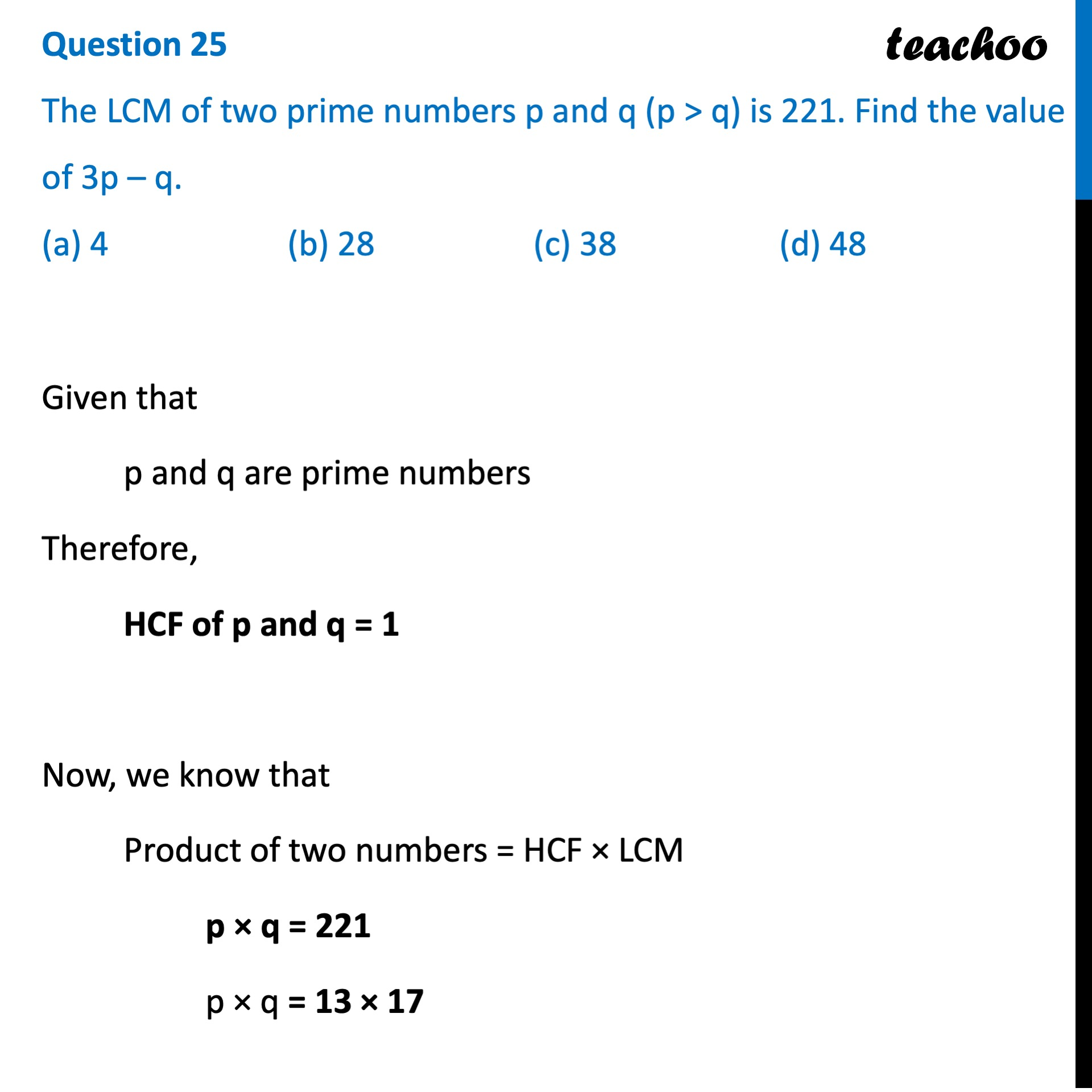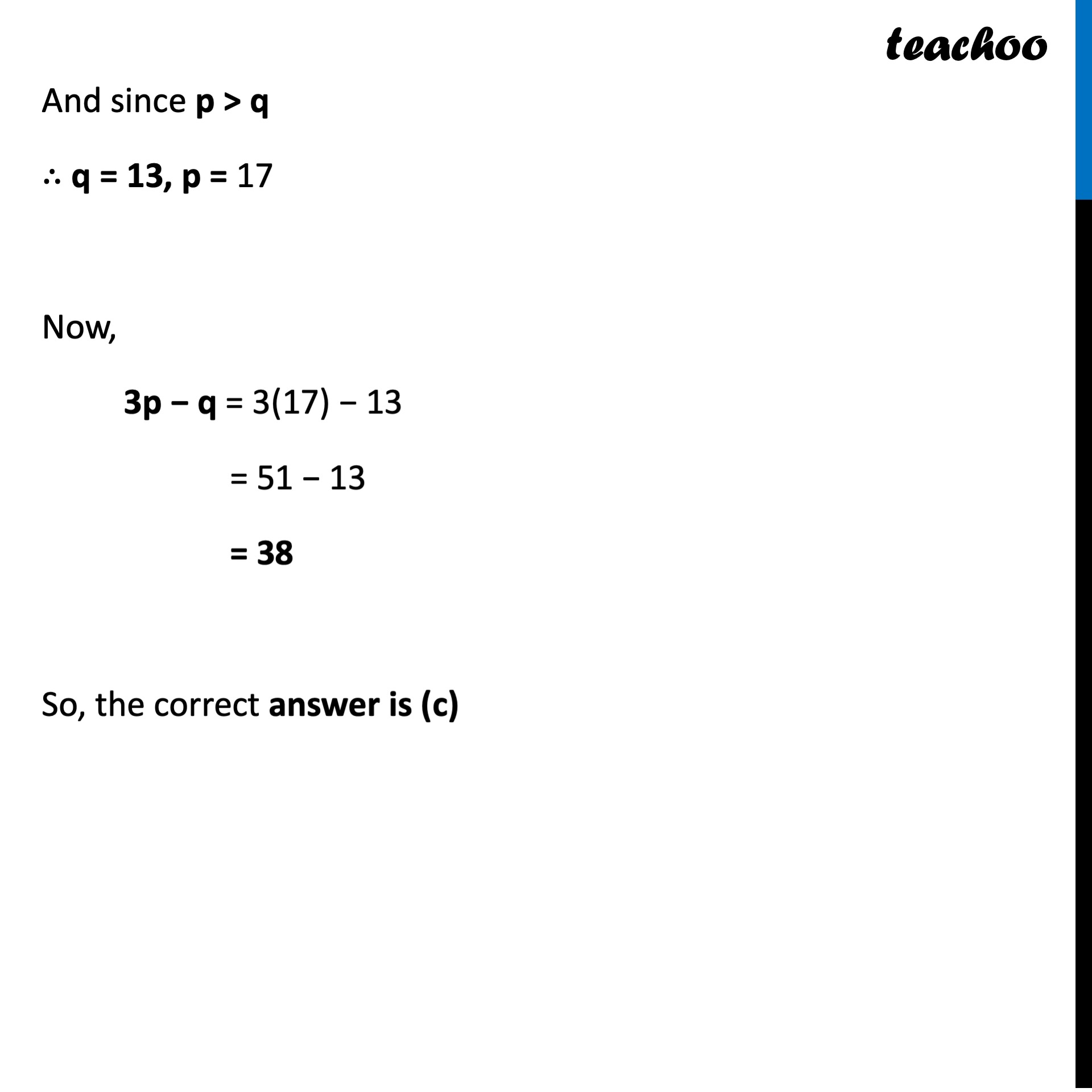## (a) 4   (b) 28   (c) 38   (d) 481. Class 10
2. Solutions of Sample Papers for Class 10 Boards
3. CBSE Class 10 Sample Paper for 2022 Boards - Maths Standard [MCQ]

Transcript

Question 25 The LCM of two prime numbers p and q (p > q) is 221. Find the value of 3p – q. (a) 4 (b) 28 (c) 38 (d) 48Given that p and q are prime numbers Therefore, HCF of p and q = 1 Now, we know that Product of two numbers = HCF × LCM p × q = 221 p × q = 13 × 17 And since p > q ∴ q = 13, p = 17 Now, 3p − q = 3(17) − 13 = 51 − 13 = 38 So, the correct answer is (c)